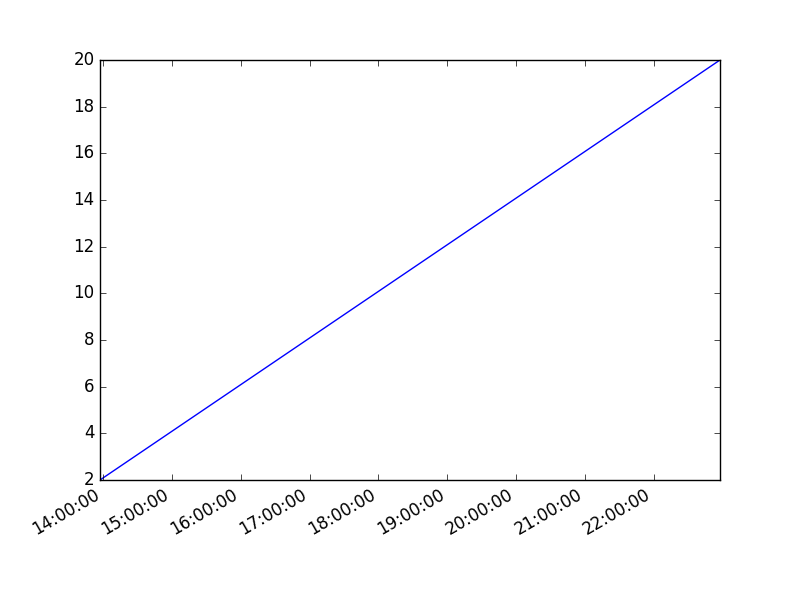# Plot time with matplotlib

Matplotlib supports plots with time on the horizontal (x) axis. The data values will be put on the vertical (y) axis. In this article we’ll demonstrate that using a few examples.

It is required to use the Python datetime module, a standard module.

Related course
Matplotlib Intro with PythonPlot time
You can plot time using a timestamp:If you want to change the interval use one of the lines below:

Time plot from specific hour/minute

To start from a specific date, create a new timestamp using datetime.datetime(year, month, day, hour, minute).
Full example: